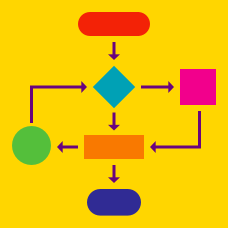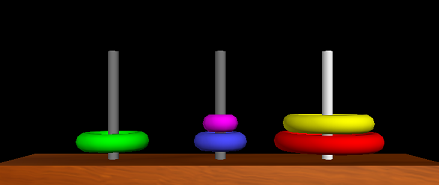Quantitative Finance

# Stacks

One impact that stacks have had in the area of computer organization is in the realization of so-called stack machines. Here, the entries in a stack are arithmetic operands and the machine instruction set contains arithmetic operators.

For example for a stack $s$, MUL is equivalent to s.push(s.pop() * s.pop()). All other elementary operations (ADD, DIV, SUB) are defined in a similar way. What will POP return once the stack machine evaluates the operations below?

 1 2 3 4 5 6 7 8 9 PUSH 5 PUSH 2 PUSH 10 PUSH 2 PUSH 9 MUL ADD DIV SUB 

The objective of Towers of Hanoi puzzle is to move all discs to stack three, obeying the following simple rules:

• Only one disk can be moved at a time.
• Each move consists of taking the upper disk from one of the stacks and placing it on top of another stack i.e. a disk can only be moved if it is the uppermost disk on a stack.
• No disk may be placed on top of a smaller disk.

We can consider each stack as a stack object. For example moving the purple disk (the top disk on the second stack) to the third stack would require the following two operations:

• stack_2.pop()
• stack_3.push(purple)

In general, moving a disk from the top of one stack to the top of another will require two operations in a row. Consider the nearly complete puzzle belowHow many operations are needed if the above puzzle is solved using the fewest number of stack operations?

Consider the standard algorithm for converting a decimal number (base 10) into a binary number (base 2). This algorithm makes use of a single stack and works by continually taking the modulo $2$ of the number and also continually dividing it by two. This allows us to finally obtain the bits of the integer.

The number of times $n$ a number has to be pushed to this stack in order to be fully converted can be expressed as a function $f(n)$. What is $f(n)$?

×